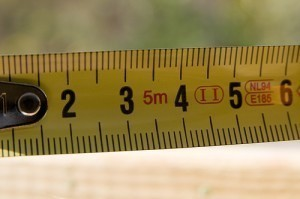# How Big is a Centimeter

A centimeter is a unit of measurement used to determine lengths of objects and is one of the most commonly used units of measurement worldwide.

These are often used to determine dress and shoe sizes, lengths of infants and toddlers as well as heights of older children and adults.

### How Big is a Centimeter?

There are approximately 2.54 centimeters in one inch while for every centimeter, there is about 0.3937 of an inch. One meter on the other hand, has one hundred centimeters while one millimeter is equivalent to about one cubic centimeter.

Further, one foot is about equivalent to 30.48 centimeters while one yard is approximately 91.44 in centimeters.

### Centimeter Fun Facts

1. Your average button found in blouses and shirts is approximately one centimeter wide.

2. The black keys on a standard piano are also about one centimeter wide.

3. Centimeters are used by most sewers and tailors as these give them more precise measurements as compared to other units of measurements used for lengths.

4. To measure snowfall, centimeter is used while rainfall is usually measured in millimeters.

5. Square centimeter means a square object is one centimeter on each of its side.

6. To convert centimeters to inches, multiply the centimeter value by .39.

7. One mile is equivalent to approximately 160,000 centimeters.

8. A person’s nails grow about 2 centimeters per year.

9. A person’s hair grows about 12.7 centimeters per year.

10. Upon returning from space, an astronaut is about 5.08 centimeters taller because the spine expands in zero gravity.11. Every year, Hawaii moves towards Japan by 10.16 centimeters.

12. Sarawak Chamber found in Malaysia is considered as the largest cave around the world and is more than 7000 centimeters in height, 98000 centimeters wide.

13. The giant Arctic jellyfish has tentacles that can extend up to 3660 centimeters in length.

14. The Hubble Space Telescope is 1310 centimeters long or about 13.1 meters.

15. The Stegosaurus measures about thirty feet in length or around 914.4 centimeters. Ironically, its brain is only as big as a walnut.

16. Motor neurons are the longest cells in a person’s body. It is located in the lower part of the spine and goes all the way down to the big toe. It is approximately 137.16 centimeters long.

17. The giant salamander, considered as the largest amphibian in the world, can grow up to five feet long or about 152.4 centimeters.

18. The average iguana measures around 10 centimeters long up to 201 centimeters.

19. The bird laughing kookaburra can grow up to 48 centimeters tall.

20. The smallest kingfisher is the African dwarf kingfisher. It can grow up to 10 centimeters only.